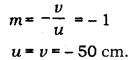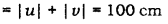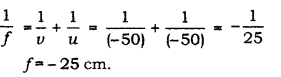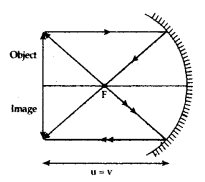# A spherical mirror produces an image of magnification on a screen placed at a distance of 50 cm from the mirror

A spherical mirror produces an image of magnification on a screen placed at a distance of 50 cm from the mirror.

1. Write the type of mirror.
2. Find the distance of the image from the object.
3. What is the focal length of the mirror?
4. Draw the ray diagram to show the image formation in this case.

1.As magnification is negative, the image formed is real.
Hence, it is a concave mirror.

2.Distance of the image from the object3.Using mirror formula, we have,4.